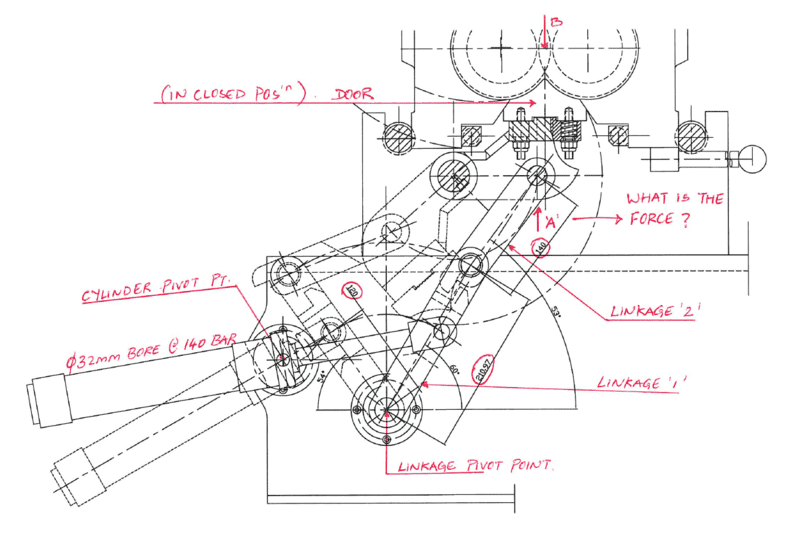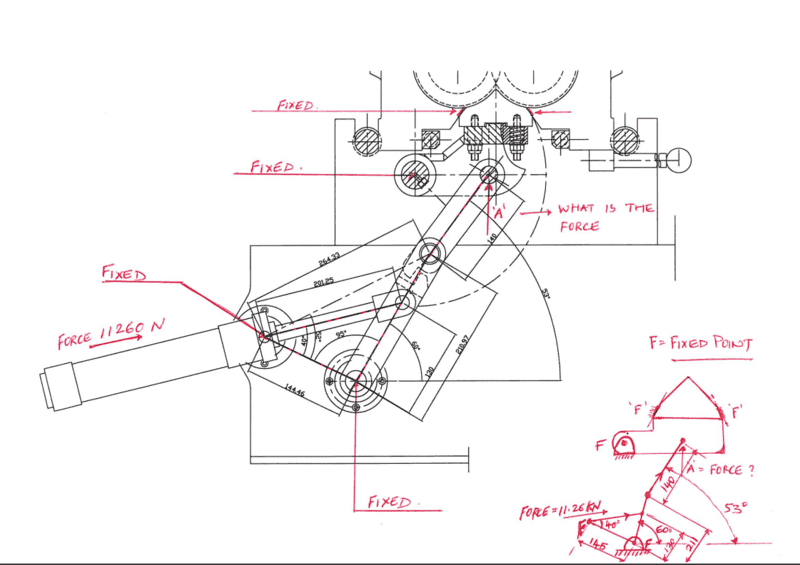# How to determine the force on the linkage

• sach2606
In summary, you are looking for the force at point 'A' when the door is in closed position. You believe that there will be some force losses due to the linkages at point 'A'.

#### sach2606

hi
Wondering if someone could help.
I need to know how to work out the the force at point 'A' ( see sketch attached).
Its a door opening and closing mechanism and it consists of a hydraulic cylinder 32mm dia bore operating at 140 bar. The door is opened and closed via couple of linkages as the cylinder opens and retracts.

I presume there will be some force losses due to the linkages at point 'A'. I am struggling to work out what will be the force at 'A' when the door is in closed position to ensure we don't put enough force at point 'B' for it to open.

Your help will be much appreciated.#### Attachments

I can't get very far with this, because I can't work out what the pivot point is for the door, nor where the fixed points are for attachment to the frame.
I think you should sketch a simple diagram of the linkage in the position you want, showing the door and frame.
Label any forces you know, then use simple mechanics, mainly moments I'd think here, to work through the mechanism.

To me, you seem to have an ill-conditioned problem. Linkage 1 and 2 are nearly straight in line when the door is closed.
When they are exactly in line, the end force will be indeterminate, unrelated to any turning force.
As they approach that point, the force can rise indefinitely for a tiny change in angle. The door would be locked by this mechanism if it passed straight.

For your open position, you are slightly further away from having two straight linkages, but not far.

hi
Thank you for the response, much appreciated.
I have a sketch as attached showing the fixed positions, I hope it makes sense.
Basically the cylinder exerts a force of 11.26kn on the first link which in turns holds the door in closed position via the second link.

All I want to know is how to work out the correct force at point 'A'?#### Attachments

Try working out some torques and forces, starting with the one you know.

The latching mechanism appears to be the insertion of the inverted V-shaped part between two rollers; is this correct? What is the application of this mechanism? It looks more like an automotive door latch than something I would expect to find in a lab.

I work problems of this sort all the time, and I find the method of virtual work to be the best approach most of the time. Have you tried that?

## 1. What is a linkage?

A linkage is a mechanical system consisting of two or more connected objects that transmit force and motion between them.

## 2. How do I determine the force on a linkage?

The force on a linkage can be determined by using Newton's laws of motion, which state that the sum of all forces acting on an object is equal to its mass times its acceleration. This can be applied to each individual component of the linkage to calculate the total force.

## 3. What factors affect the force on a linkage?

The force on a linkage can be affected by various factors, such as the weight and mass of the objects connected by the linkage, the angle and direction of the force being applied, and any external forces acting on the system.

## 4. Can I use mathematical equations to determine the force on a linkage?

Yes, mathematical equations such as the equations of motion and the law of conservation of energy can be used to determine the force on a linkage. These equations take into account the various factors that affect the force on the linkage.

## 5. How can I ensure accuracy when calculating the force on a linkage?

To ensure accuracy when calculating the force on a linkage, it is important to accurately measure and record all the relevant factors, such as the mass and weight of the objects, the angle and direction of the force, and any external forces. It is also important to use appropriate equations and units for the calculations.